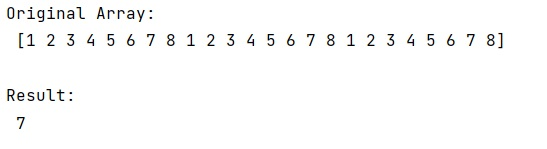# How to get the index of a maximum element in a NumPy array along one axis?

Learn, how to get the index of a maximum element in a NumPy array along one axis?
Submitted by Pranit Sharma, on December 26, 2022

NumPy is an abbreviated form of Numerical Python. It is used for different types of scientific operations in python. Numpy is a vast library in python which is used for almost every kind of scientific or mathematical operation. It is itself an array which is a collection of various methods and functions for processing the arrays.

## Getting the index of a maximum element in a NumPy array along one axis

Suppose we are given a multi-dimensional NumPy array and we need to get the maximum values over the axes.

For this purpose, we need to use the numpy.argmax() method which returns the index of the maximum element along the row.
To let the compiler know that we want the index of the maximum element along the rows of the array, we need to pass the argument as 'axis=0'.

Let us understand with the help of an example,

## Python code to get the index of a maximum element in a NumPy array along one axis

```# Import numpy
import numpy as np

# Creating a numpy array
arr = np.array([1,2,3,4,5,6,7,8,1,2,3,4,5,6,7,8,1,2,3,4,5,6,7,8])

# Display original array
print("Original Array:\n",arr,"\n")

# Index of maximum element
res = arr.argmax(axis=0)

# Display the result
print("Result:\n",res,"\n")
```

Output: